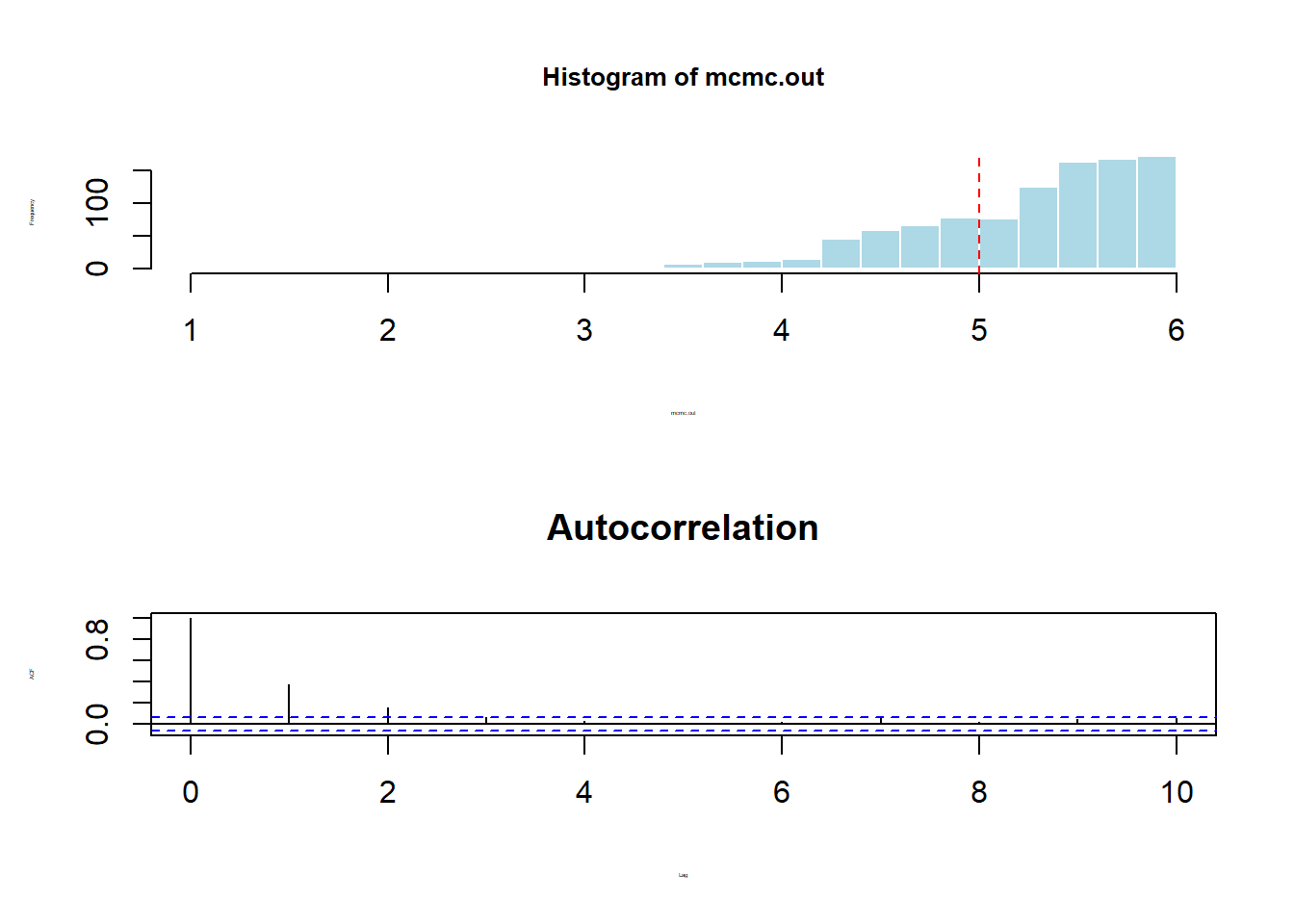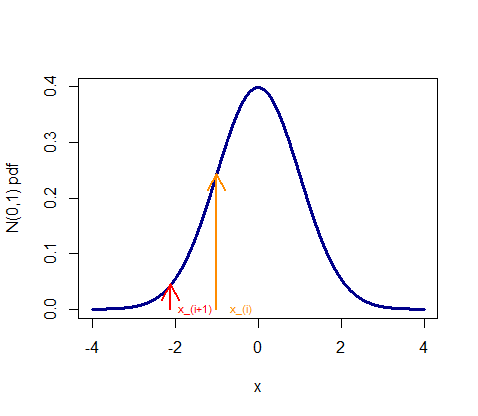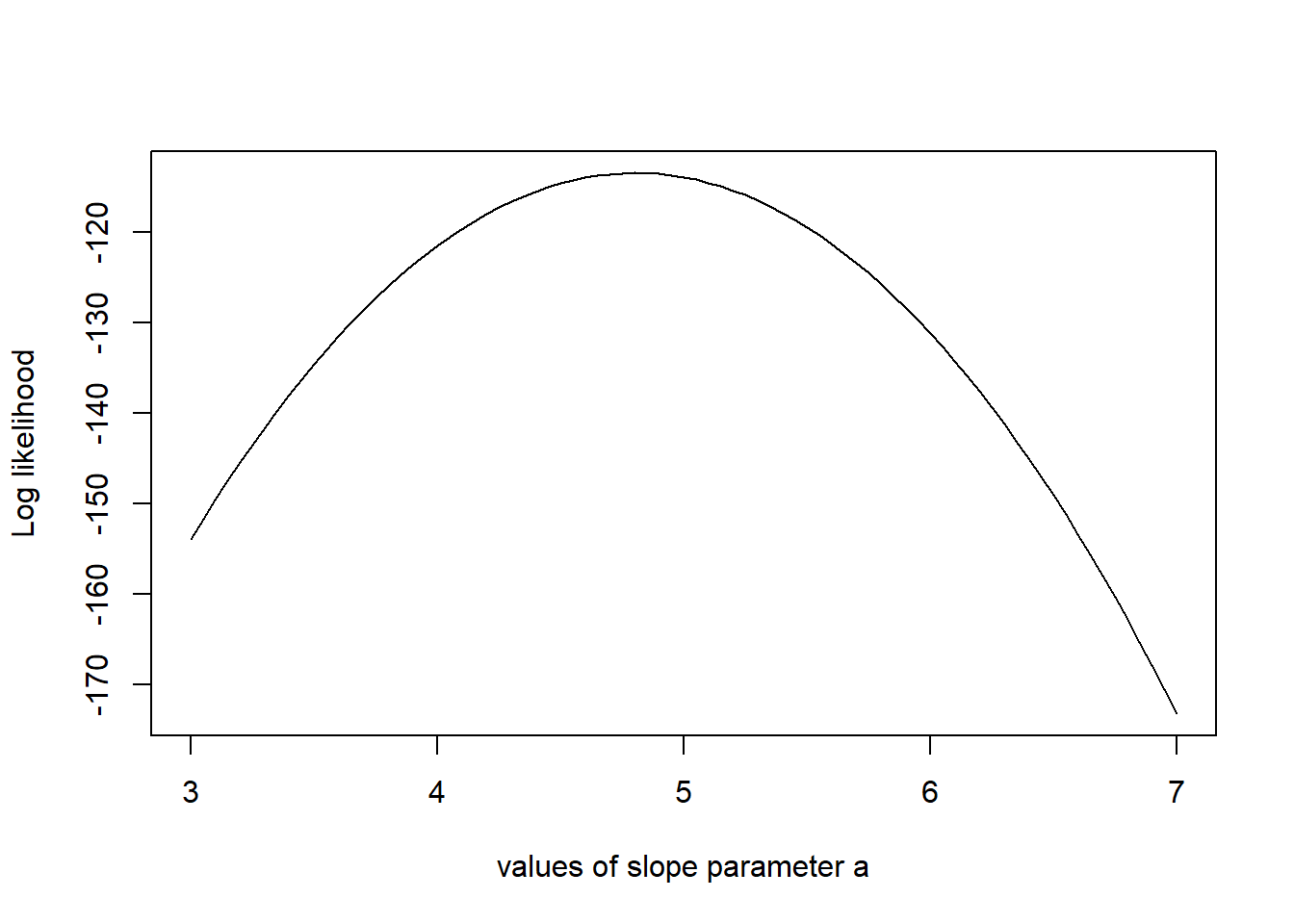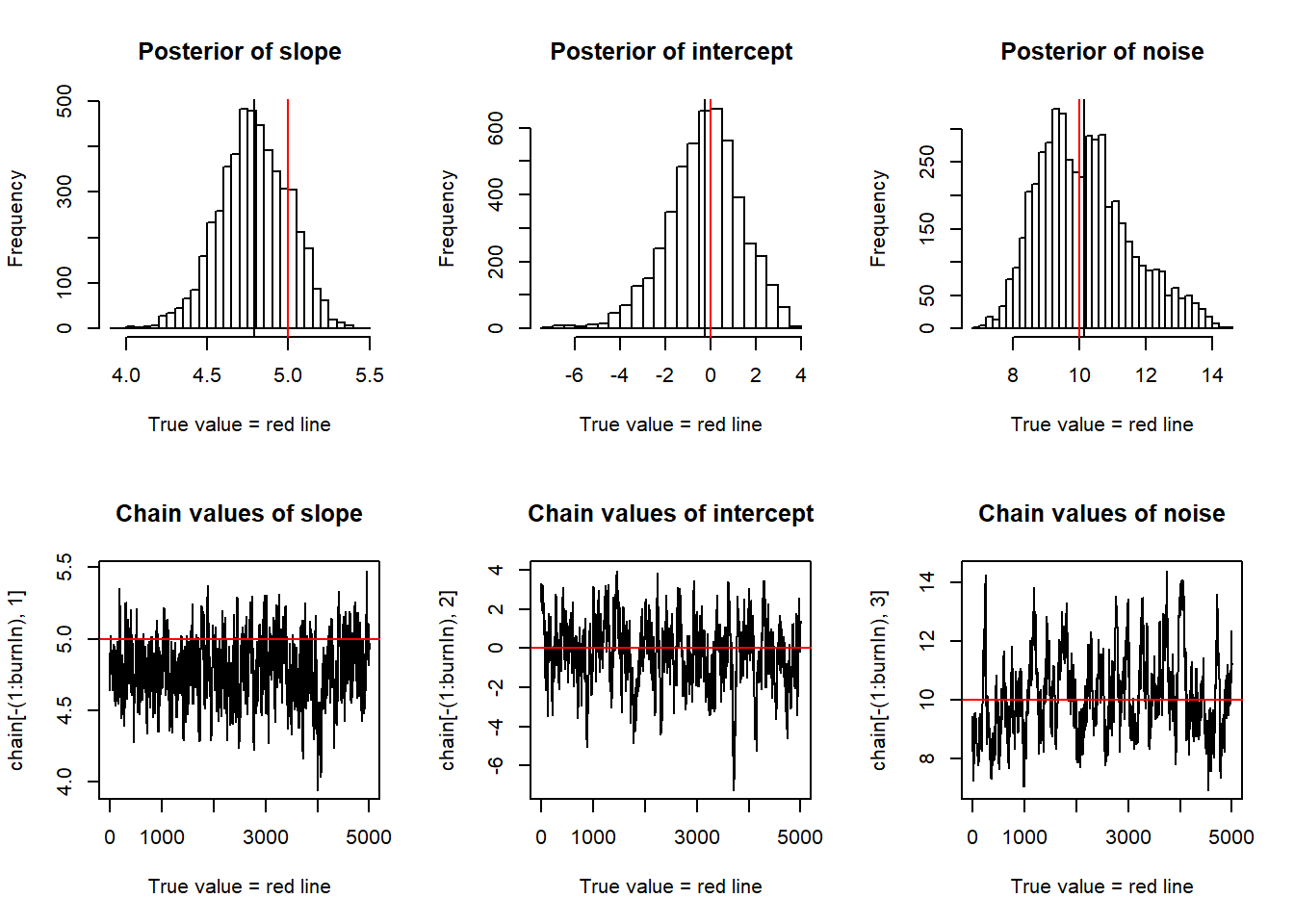### MARKOV CHAIN MONTE CARLO:

OBJECTIVE: Find the expected value and value range (CI) of a high-dimensional pdf. We perform a random walk throught the pdf, favoring values with high “probability” (high density values). From a starting point, we pick a nearby point and its probability is higher, we move there; otherwise, we stay put. If we carry this long enough we hit every point in the manifold with a frequency proportional to its probability (ergodicity).

There are different uses of MCMC, but the examples below reflect two different settings:

1. We are sampling from a posterior distribution (Bayesian setting): The parameter space we are sampling from is the posterior distribution parameters $$\Theta$$, e.g. the mean of a specific distribution in the case of a single parameter, and we sample in such a way that each point is selected with a frequency proportional to the value of the distribution (or an un-normalized function).

The context for this problem is a Bayesian function of the form

$f_\Theta(\Theta=\vec \theta\vert D)=\frac{f_X\left(X=D\vert \Theta\right)\,f_\Theta\left(\Theta=\vec \theta\right)}{\int\int\int f_X\left(D\vert \Theta\right)\;f_\Theta\left(\Theta=\vec \theta\right)\;d\theta_1\cdots d\theta_n}$ with an unsolvable denominator. In this case, if we can’t use direct simulation, the inverse of the inverse cdf method, or the accept-reject method, we can sample from an un-normalized function, i.e. leaving aside the denominator, which is meant to normalize the pdf on the LHS of the equation. At every step, a proposal distribution will result in a new $$\Pr(\text{likelihood})\times\Pr\text{(prior)}$$ calculation, which will be accepted provided that it is higher than the prior calculated value (or proportional its value).

An example of sampling the parameters of truncated normal distribution, $$\mathcal N(5,3)\,\mathbb 1[1<x<6]$$ between 1 and 6 (in this case without data - no Bayes’ equation) is presented.

The idea is to use an un-normalized target density, $$f(\theta) = \exp{-\frac{(x-\mu)^2}{2\sigma^2}}.$$ Each value sampled along the simulation, $$\theta^{(i)}$$ is contraposed to a value extracted from the proposal distribution, $$q(\theta^*\vert \theta^{(i)}),$$ that depends on $$\theta^{(i)}$$ (autoregressive series).

After a proposed value is put forward, an acceptance probability is calculated with the formula:

$\rho(\theta^{(i)},\theta^*)=\min\left\{ 1, \frac{f(\theta^*)}{f(\theta^{(i)})}\frac{q(\theta^{(i)}\vert \theta^*)}{q(\theta^*\vert\theta^{(i)})} \right\}$

where $$\frac{f(\theta^*)}{f(\theta^{(i)})}$$ is the ratio of the target of the unnormalized target density at the proposed value over the height of the density at the current value; and $$q(\theta^{(i)}\vert \theta^*)$$ is the probability of getting to $$q(\theta^{(i)}$$ from $$q(\theta^*)$$, while $$q(\theta^*\vert\theta^{(i)})$$ is the probability of getting to $$q(\theta^*)$$ from $$q(\theta^{(i)}.$$

In a random walk Metropolis Hasting algorithm, $$q(\theta^{(i)}\vert \theta^*=q(\theta^*\vert\theta^{(i)}$$ the proposal is symmetrical and the expression above is simplified to

$\rho(\theta^{(i)},\theta^*)=\min\left\{ 1, \frac{f(\theta^*)}{f(\theta^{(i)})} \right\}.$

Evidently, if $$\frac{f(\theta^*)}{f(\theta^{(i)}}>1,$$ the probability of accepting the proposed value is a given $$\Pr()=1,$$ and an update will take place whereby $$\theta^{(i+1)}=\theta^*.$$ Otherwise we are left with a probability that this update happens: if a random uniform draw from $$[0,1]$$ is above $$\rho$$ the update takes place, and $$\theta^{(i+1)}=\theta^*,$$ but if $$\text{runif}(0,1)<\rho,$$ the update does not take place, and $$\theta^{(i+1)}=\theta^{(i)}.$$

# MCMC of Truncated normal (5, 3) between 1 and 6.

set.seed(12)

metropolis1 = function(n = 1000){
## SO WE FEED A NUMBER OF ITERATIONS

vec = vector("numeric", n) # Start a numeric vector of length n

mean = 5        # Mean of the posterior we want to sample from.
vec = mean   # The first entry of the vector is 5.
SD=3            # SD of the posterior

for (i in 2:n) {# The others entries in the vector "vec" (after the first) are....

innovation = rnorm(1, 1, 1) # The proposal distribution
# The values of vec are updated by adding an "innovation" sampled from a symmetrical distr.

candidate_value = vec[i - 1] + innovation # whatever the value was on prior round + rnorm
# THE CANDIDATE (PROPOSED VALUE) COMES FROM A RANDOM NORMAL, but carries on the info
# from the prior value (it's an autoregressive time series)

while(candidate_value < 1 || candidate_value > 6)
{inn = rnorm(1, 1, 1); candidate_value = vec[i - 1] + inn } # But it is a truncated normal!

acceptance_ratio = min(1, exp(-(candidate_value - mean)^2 / (2*SD^2))/
exp(-(vec[i - 1] - mean)^2 / (2*SD^2)))

# since we pretend not to have the normalizing denominator, we don't use dnorm().

ifelse(runif(1) < acceptance_ratio, vec[i] <- candidate_value, vec[i] <- vec[i - 1])

# Here we take a random uniform, this time from 0 to 1.
# and only if the ratio is larger than it will we replace
# the prior x with the candidate value.
}
vec
}

mcmc.out = metropolis1(1e3)
par(mfrow = c(2,1))
hist(mcmc.out,30,col="lightblue",border=F,cex.main=.8, cex.lab=.2)
abline(v=5, lty=2, col=2)
acf(mcmc.out, lag.max=10, main="Autocorrelation", cex.lab=.2)summary(mcmc.out)
##    Min. 1st Qu.  Median    Mean 3rd Qu.    Max.
##   1.165   4.893   5.402   5.245   5.704   6.000

The AR series pattern is evident in the ACF(mcmc.out) plot.

1. In other cases the output is a value, not a pdf. We use observed data (for instance, atmospheric measurements) to construct a likelihood function which is maximized by the model output. An easy choice is the exponential decay function $$\exp(-\text{cost}$$ with $$\text{cost}=\Vert \text{model output - observed data}\Vert.$$ The target density is replaced by the likelihood function. The proposed density are random steps chosen uniformely between boundaries.

From this post:

Another daunting issue in statistics. The question is old, but the introductory examples on-line are hard to come by. So let me simplify two great examples just in case someone following the Markov random walk of PageRank lands here befuddled by MCMC, and full of anticipation for an easy to follow answer. How likely? That could be a follow-up question.

FIRST EXAMPLE:

The post recreates a standard normal distribution $$N(0,1)$$ using the Metropolis - Hastings algorithm. Far from a challenging case but a good one to dissect. I have collected the code from the post here for convenience and for annotations.

The difficulty is in realizing that after going through all the mechanical steps, there is just one magical trick: the binary decision of accepting or rejecting a proposed value.

It’s good to keep in mind that what we want is to generate a bunch of values ($$x$$) that when plotted on a histogram look like a bell curve, centered (mean) at $$0$$ and with a standard deviation (sd) of $$~ 1$$. The problem is that we don’t have predetermined parameters; that would be too easy, simply running something along the lines of rnorm(10000).

We do so by taking tiny (or larger, it won’t change the results too much) random stochastic steps through the use of pseudorandom number generators. In the example, the magnitude of the step is set by eps, which determines the $$\epsilon$$, or size of the step to the left or to the right from the previous accepted value ($$x_i$$). This will be the starting line in the process that generates a new proposed value to fill in the next “link” of the “chain”, $$x_{i+1}$$. OK, I’m talking about generating runif(1, - eps, eps) to either subtract or add to $$x_i$$.

Every proposed value would thus differ from the prior value in a random fashion, and within the boundaries of [- eps,+ eps].

Enter the Markov kernel. We need to assess the probability of transitioning to the new proposed value somehow, much like in a transition Markov matrix on a given state at time $$i$$ tells about the probability of the new state at time $$i + 1$$. If in doubt go here, or ask the grandma who’s been paying attention to all the “How would you explain quantum physics to your nanna?” type of questions (don’t the matrices look nice? ;-) ).

To get this probability of acceptance into the bouquet of culled values, we simply compare the height of the $$N(0,1)$$ density at the proposed new value ($$x_{i+1}$$) to the previous (already accepted value), ($$x_{i}$$), just like this:And we take the ratio of both values: min(1, dnorm(candidate_value)/dnorm(x)). Since we want a probability, the resultant calculation cannot go over $$1$$, which occurs whenever the $$N(0,1)$$ $$pdf$$ at $$x_{i+1}$$ (candidate value) is greater than at $$x_i$$, amounting to automatic acceptance of the proposed value, and explaining the min(1, ...) part of the code. Otherwise, the closer the dnorm value of the proposed value is to the previous value, the higher the odds of it being accepted.

So we do have a probability of acceptance, but we need to make a binary decision (accept the new proposed value, or reject it). And here comes the magic trick: if the probability calculated as min(1, dnorm(candidate_value)/dnorm(x)) is greater than a runif(1) uniform draw from $$0$$ to $$1$$ (as close as it gets to a coin toss for a continuous value), we accept, and fill in the x[i+1] entry of the chain with the proposed value; otherwise, we fill it in with a repeat of the previous value, x[i]… The idea would be, better two of the same than one too far away into the tails.

We do this thousands of times and we collect all these values (only the accepted and repeated values), and when we plot the histogram, we get a nice normal curve with a sd close to $$1$$, and centered at $$0$$.

Just one final point: Where do we start? Probably not to relevant, but in the simulation, we fill in the first value as $$0$$, x = 0; vec = x before looping through all the rest of iterations, and let the process follow its course.

SECOND EXAMPLE:

This is more exciting, and makes reference to estimating the parameters of a linear regression curve by calculating log likelihoods for random parameters given a dataset. However, the exegesis of the code lines is built in the condensed simulation saved here, following very similar steps to the first example.

# MCMC Monte Carlo Markov Chains from
# https://theoreticalecology.wordpress.com/2010/09/17/metropolis-hastings-mcmc-in-r/

set.seed(123)

## THE TRUE DATA:

slope <- 5          # This is the slope
intercept <- 0      # This is the intercept
noise<- 10          # This is the noise
sampleSize <- 31

# create independent x-values

x <- (-(sampleSize-1)/2):((sampleSize-1)/2)

# As many (x,y) points as the sampleSize

# create dependent values according to ax + b + N(0,sd):

y <-  slope * x + intercept + rnorm(n=sampleSize, mean=0, sd = noise)

## LIKELIHOOD FUNCTION:

likelihood <- function(param){

a =  param # First column for the specified row of "chain" (below), corresponding to the slope
b =  param # Second column ..., corresponding to intercept.
sd = param # Third column ..., corresponding to sd.

pred = a * x + b # predicted

# We use a, b, sd to generate a predicted value with a line function.

singlelikelihoods = dnorm(y, mean = pred, sd = sd, log = T)

# And we see how "tall" the value corresponding to the defined (above) true (data) y's would be if the predicted
# value was the mean - we are really measuring in a way the residual.
# with homoskedesticity there is now a curve of errors distributed normally around 'pred'

# This tells us how far the true proposed y is from the true y.

sumll = sum(singlelikelihoods)
# Now we sum because the LOG likelihood function of independent y values is added, as much as the likelihood
# without log would be multiplied.
# So we are getting a likelihood measure for entire set of y values.

return(sumll)
}

# How does the likelihood of the data change depending on the slopes:

slopevalues <- function(x){return(likelihood(c(x, intercept, noise)))}

slopelikelihoods <- lapply(seq(3, 7, by=.05), slopevalues)
plot (seq(3, 7, by=.05), slopelikelihoods , type="l",
xlab = "values of slope parameter a",
ylab = "Log likelihood")# Prior distribution

prior <- function(param){
a =  param
b =  param
sd = param
aprior =  dunif(a, min=0, max=10, log = T)
# We calculate the probability of the slope based on a uniform from 0 to 10.
bprior =  dnorm(b, sd = 5, log = T)
# We calculate the prior probability of the intercept based on normal with sd of 5.
sdprior = dunif(sd, min=0, max=30, log = T)
# ... and the prior of the noise on a uniform from 0 to 30.
return(aprior + bprior + bprior)
# The probability of these three values would have to be multiplied, if it weren't for "log = T".
}

posterior <- function(param){
return (likelihood(param) + prior(param))
}

# The param are going to be rows in the "chain" (see below), corresponding to slope / intercept / noise (sd).
# Since both the prior likelihood and the likelihood itself are log-ed, we can add their values.

######## Metropolis algorithm ################

proposalfunction <- function(param){
return(rnorm(3, mean = param, sd= c(0.1,0.5,0.3)))
}

# Takes in three means (corresponding to a row of "chain" and generates three random numbers (a proposed new row for chain).

run_metropolis_MCMC <- function(startvalue, iterations){

chain = array(dim = c(iterations + 1, 3))

# array creates this:

#         [,1] [,2] [,3]
# [1,]     NA   NA   NA
# [2,]     NA   NA   NA
# ...
#   NA   NA   NA                          for iterations = 10000

chain[1,] = startvalue

# startvalue fills in first row.

for (i in 1:iterations){

proposal = proposalfunction(chain[i,])

# we run a function within run_metropolis_MCMC through iterations.
# The proposalfunction was already defined above.
# It takes the three values of the row at time i and uses them as means in the proposal function,
# which produces this for i = 1:

# chain[1,]
#   4    0    10
# proposalfunction <- function(param){ # Redefining the function to see what it does with rows of chain.
# set.seed(0); return(rnorm(3, mean = param, sd= c(0.1, 0.5, 0.3)))
# }
# proposalfunction(chain[1,])
#   4.1262954 -0.1631167 10.3989398
# proposalfunction(c(4, 0, 10)) # So it uses the three valuese of each column of chain as means to generate random
# normals (3 of them) with different standard deviations - since in this case the mean of the columns of chain
# go from ~ 5 for the slope to 0 for the intercept to 10 for the noise, using these values as means to generate
# randoms will end up in similar rows:
#   4.1262954 -0.1631167 10.3989398

# So basically three similar, but not equal values to the row i.

probab = exp(posterior(proposal) - posterior(chain[i,]))

# Now we calculate the difference in the posterior probability of the proposed parameters with respect to
# the parameters in row i.

if (runif(1) < probab){
chain[i + 1,] = proposal
}else{
chain[i + 1,] = chain[i,]
}
}
# and we toss a coin with the runif. If the difference is greater than the runif the proposal will occupy the next row
# otherwise, we duplicate the row i to fill in row i + 1.
return(chain)
}

startvalue = c(4, 0, 10)
# The startvalue is the first row of the chain. 4 above goes to slope; 0 to intercept, and 10 to noise or sd.

chain = run_metropolis_MCMC(startvalue, 10000)

# The object chain will be the result of running "run_metropolis_MCMC 10,000 times.

#chain
#                 [,1]                 [,2]                  [,3]
#[1,]          4.000000             0.00000000             10.00000
#[2,]          4.000000             0.00000000             10.00000
# ...
# ...
#[10000,]      4.980106             1.2658856              11.19833
#[10001,]      4.930751             1.3969398              11.24195
#              ~ 5 (slope)          ~ 0 (intercept)        ~ 10 for noise

burnIn = 5000

# The first 5000 rows of proposed values will be thrown out to collect simulations after reaching a steady-state.

acceptance = 1 - mean(duplicated(chain[-(1:burnIn),]))

# chain[-(1:burnIn),] selects all the rows of "chain" excluding rows 1 through burnIn, which is 5000. So it results
# in 5001 rows with three columns.
# the function duplicated is boolean, and looks for duplicated values in the rows.
# mean(...) is the percentage of duplicated rows from 5001 to 10001.
# and 1 - (...) is the acceptance rate for the proposed new values.

### Summary: #######################

# Plotting of the slopes with mean and true value:

par(mfrow = c(2,3))
hist(chain[-(1:burnIn), 1], nclass=30, , main="Posterior of slope", xlab="True value = red line" )
abline(v = mean(chain[-(1:burnIn), 1]))
abline(v = slope, col="red" )

# Plotting of the intercepts with mean and true value:

hist(chain[-(1:burnIn),2], nclass=30, main="Posterior of intercept", xlab="True value = red line")
abline(v = mean(chain[-(1:burnIn),2]))
abline(v = intercept, col="red" )

# Plotting of the noise with mean and true value

hist(chain[-(1:burnIn),3],nclass=30, main="Posterior of noise", xlab="True value = red line")
abline(v = mean(chain[-(1:burnIn),3]) )
abline(v = noise, col="red" )

# Time sequence of the slopes:
plot(chain[-(1:burnIn), 1], type = "l", xlab="True value = red line" , main = "Chain values of slope", )
abline(h = slope, col="red" )

# Time sequence of intercepts:
plot(chain[-(1:burnIn),2], type = "l", xlab="True value = red line" , main = "Chain values of intercept", )
abline(h = intercept, col="red" )

# And noise:
plot(chain[-(1:burnIn),3], type = "l", xlab="True value = red line" , main = "Chain values of noise", )
abline(h = noise, col="red" )# for comparison these are OLS values for slope and intercept based on the data (not MCMC):
summary(lm(y~x))
##
## Call:
## lm(formula = y ~ x)
##
## Residuals:
##      Min       1Q   Median       3Q      Max
## -18.9593  -6.6725  -0.6956   6.4944  18.1874
##
## Coefficients:
##             Estimate Std. Error t value Pr(>|t|)
## (Intercept)  -0.3183     1.7391  -0.183    0.856
## x             4.8057     0.1944  24.715   <2e-16 ***
## ---
## Signif. codes:  0 '***' 0.001 '**' 0.01 '*' 0.05 '.' 0.1 ' ' 1
##
## Residual standard error: 9.683 on 29 degrees of freedom
## Multiple R-squared:  0.9547, Adjusted R-squared:  0.9531
## F-statistic: 610.9 on 1 and 29 DF,  p-value: < 2.2e-16